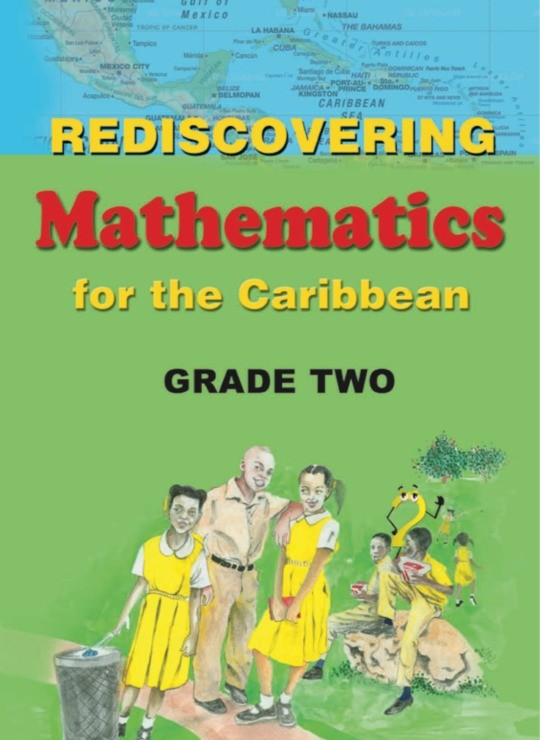\$8.99

# Rediscovering Mathematics for the Caribbean: Grade 2

US\$ 8.99
The publisher has enabled DRM protection, which means that you need to use the BookFusion iOS, Android or Web app to read this eBook. This eBook cannot be used outside of the BookFusion platform.
Book Description

Rediscovering Mathematics for the Caribbean: Grade Two, is the fourth of a comprehensive series of eight textbooks which covers the requirements of the Mathematics syllabuses for Primary Schools in the Caribbean. The text provides a firm foundation in Mathematics for primary school pupils utilizing a learner-centred approach.

Some Key Features:

Basic numeracy skills are emphasized and topics are carefully introduced.
Numerals, exercises and illustrations provide pupils with a lot of practice, thereby enhancing their understanding of mathematical concepts.
Concepts are expressed in simple, clear and easy to understand language that is appropriate to the grade level and subject.
Meaningful connections are made to real life situations.
Other features include activities that provide opportunities for students to engage in critical thinking and problem solving, and students are required to perform tasks that extend and reinforce knowledge and skills learnt. The language used throughout the series is simple and straightforward and pupils and teachers should find the learning and teaching experience fun and quite enjoyable.

• Cover Page
• Title Page
• Copyrright Page
• Acknowledgement
• Preface
• Contents
• Counting Chart
• I Can Count
• Review Of Numerals And Their Number Names: 0 – 30
• Vamos A Contar En El Español
• Get Ready To Check Yourself
• Sets
• How Many?
• Let Us Compare
• Equivalent Sets
• Comparing Sets
• More Than, Greater Than
• Is More Than/Is Greater Than ‘>’
• Is Less Than ‘<’
• ‘=’ Is Equal To
• Measurement
• The Calendar
• Which Is Heavier? Which Is Lighter?
• I Can Count
• Numerals And Their Number Names: 3 0 – 100
• Counting
• Counting By 2s
• Counting By 3s
• Counting By 4s
• Counting By 5s
• Showing Addition Using The Number Line 0 – 20
• Ordinal Numbers
• Comparing
• Gathering Data
• Tallying
• Pictographs
• I Can Count
• Numerals: 100 – 500
• Measurement
• The Thermometer
• Are We Odd or Even?
• Measurement
• Measuring Lengths Using The Centimetre And The Metre
• Estimate And Verify
• Count In Tens
• Number Order
• Measurement
• Mass
• Comparing
• Grams/Kilograms
• Which One Is Heavier?
• Place Value
• Addition Of Tens And Ones
• Expanded Notation
• Expanding Numbers
• Measurement
• Capacity: Litres
• Measuring In Millilitres And Litres
• Money
• Jamaican Money
• Amounts Of Money
• Let’S Go Shopping
• Measurement
• Telling Time
• By The Hour
• Telling Time At Half Hour
• Money
• Subtraction Of Money
• Shopping
• Subtraction
• Subtraction, Take Away, Minus, Finding The Difference
• Subtracting (Taking Away) Using The Number Line 0 – 20
• Division
• Learning To Share Equally
• I Can Count
• Numerals 500 – 800
• 2 Digit Numbers To 3 Digit Numbers (Without Renaming)
• Addition Of 3 Digit Numbers (Without Renaming)
• Addition Of 2 Digit Numbers To 3 Digit Numbers (Renaming)
• Adding 3 Digit Numbers (Renaming)
• Multiplication
• Grouping
• Groups Of 2
• Multiplying 2 Digits And 1 Digit Numbers By 1 Digit Numbers (Without Renaming)
• Multiply 2 Digits And 1 Digit Numbers By 1 Digit Numbers. (With Renaming)
• Measurement
• Telling Time At Quarter Past The Hour
• At Quarter To The Hour
• Fractions
• The Half (1/2)
• One Quarter 1/4
• Identifying 1/2 Of A Whole And Of A Set
• Identifying 1/4 Of A Whole And Of A Set
• Thirds 1/3
• One Third (1/3) Of A Set
• Proper Fractions
• Improper Fractions
• Equivalent Fractions
• Mixed Number
• Subtracting Fractions
• Comparing Fractions
• Shapes: Flat Shapes
• Cylinders
• Cones
• Pyramids
• The Sphere
• Measurement
• Schedules/Timetables
• Minutes To The Hour
• Minutes Past The Hour
• Paths
• Shapes
• Do You Know These Shapes?
• Geometry
• Lines
• Place Value
• Hundreds, Tens And Ones
• Comparing Numbers
• Check Yourself
• Subtraction
• Subtraction
• 1 Digit Numbers From 2 Digit Numbers
• 3-Digit Numbers (No Renaming)
• Number Order
• What Are The Missing Numerals?
• I Can Count
• Numerals: 800 – 1000
• Solve The Following Problems
• Multiplication
• Arrays
• Grouping
• Multiplying 2 Digits And 1 Digit Numbers By 1 Digit Numbers
• Assessment
• Calculator
• SHAPES
• Lines Of Symmetry
• Straight Lines And Curves
• Gathering Data
• The Block Graph
• Graphs/Pictographs
• Finding The Unknown